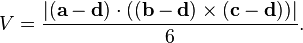## Volume of Tetrahedron/Parellelepiped Calculator

 Enter the vertex P ,, Enter the vertex Q ,, Enter the vertex R ,, Enter the vertex S ,, Volume of Parellelepiped Volume of Tetrahedron

### Volume of tetrahedron formula

The volume of a tetrahedron is 1/3 (area of the base) * height

If the tetrahedron with vertices a = (a1, a2, a3), b = (b1, b2, b3), c = (c1, c2, c3), and d = (d1, d2, d3), the volume is (1/6)·|det(a − d, b − d, c − d)|Thinkcalculator.com provides you helpful and handy calculator resources.